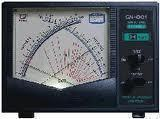•• # What is VSWR?

VSWR (Voltage Standing Wave Ratio) is a metric commonly used with antenna systems for ham or shortwave radio communication. VSWR is normally defined as a ratio with a 1:1 VSWR, indicating that there is an exact or perfect match between all antenna system elements. The VSWR can also be expressed by comparing Vmax with Vmin in a ratio.

## Why does VSWR Exist?

In order to obtain maximum power from a load, the load and generator impedance must match. If there is any mismatch or difference, then maximum power transfer does not occur. This same concept applies to antennas and transmitters. Since the antenna is normally some distance away from the transmitter, the feed line has no loss and matches both the antenna input impedance and transmitter output impedance to have a VSWR of 1:1. The resulting voltage and current would then have to be constant over the length of the feed line. If there is any deviation from this, then a standing wave of voltage and current is created and the VSWR will exceed the 1:1 ratio.## How is VSWR Measured?

VSWR and its effects can be measured in several ways, including return loss, reflected power, reflection coefficient, and transmitted power loss. All of these  measure the proportion of power that a mismatched antenna reflects to the transmitter, just in different ways. The reflection coefficient is a measurement of the feed line’s mismatch at the antenna. The following formula expresses the reflection coefficient: P =(Z1-Zo)/(Z1+Zo), where Z1 refers to the antenna impedance, Z0 refers to the feed line impedance, and all variables are complex numbers. When Z1 = Zo there is no reflected signal in the antenna system. This condition is rarely seen, however, and is used more in antenna theory than in practical antenna applications.

## Where are VSWR Measurements Made?

Along an antenna’s feed line, the voltages have a voltage minimum and maximum based on the relative phase difference of the traveling waves along the line. The minimum and maximum points normally occur ¼ wavelength apart from each other. Since the adoption of coaxial cable in antenna systems, VSWR measurements are normally made on the transmitter end of the feed line.

1. #### etta.bartlett2

26 July, 2015 at 4:16 am
462 queries in 0.497 seconds.